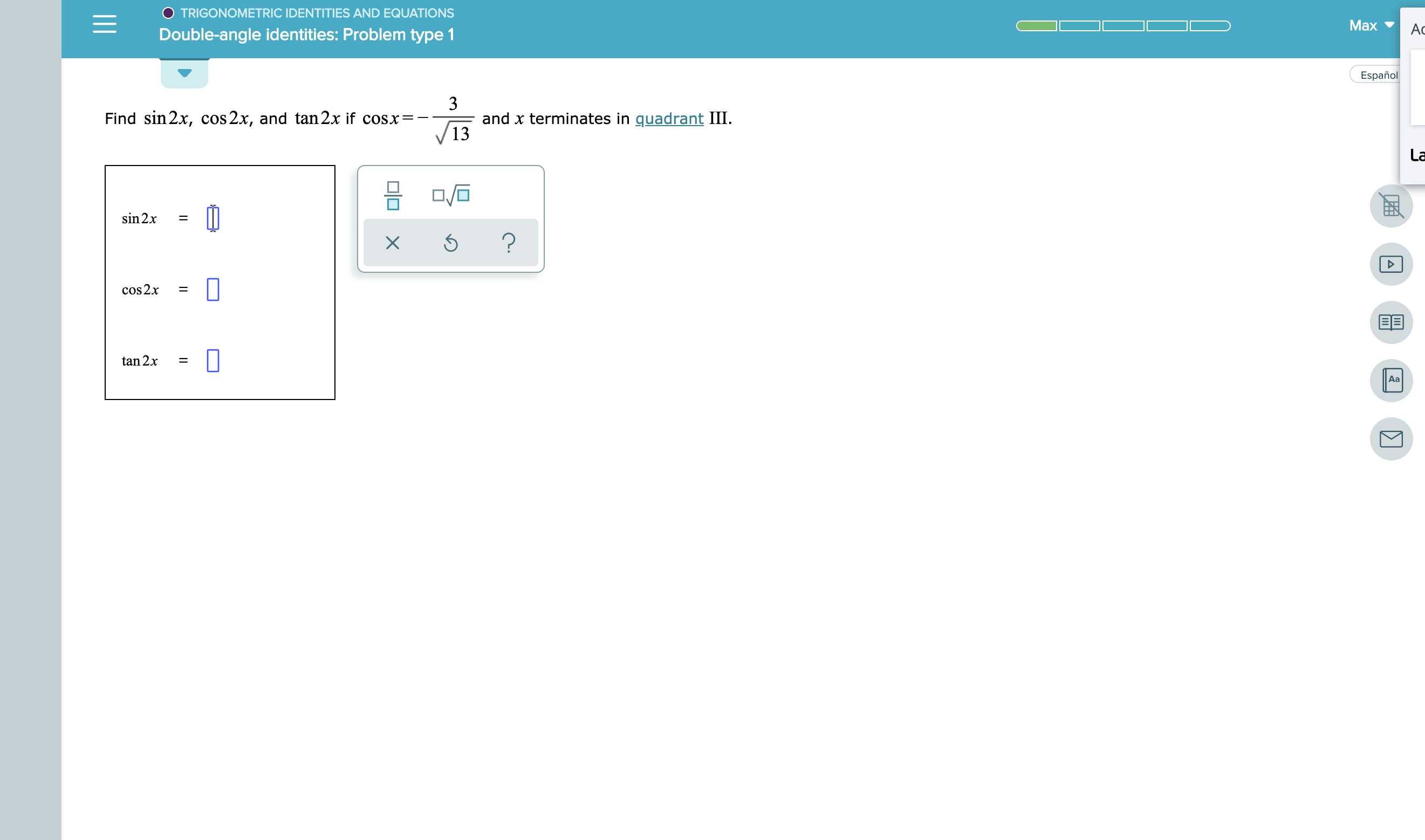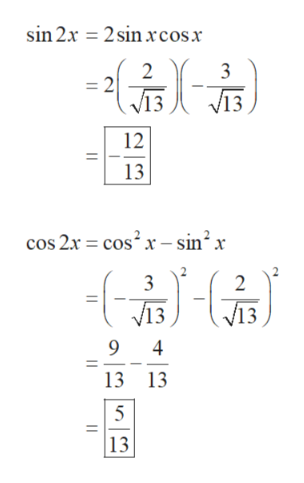TRIGONOMETRIC IDENTITIES AND EQUATIONSMaxАсDouble-angle identities: Problem type 1Español3and x terminates in quadrant IIIFind sin 2x, cos 2x, and tan 2x if cosx=-13Lasin 2x?cos2x=tan 2.xAa미미X

Question

See attachmenthelp_outlineImage TranscriptioncloseTRIGONOMETRIC IDENTITIES AND EQUATIONS Max Ас Double-angle identities: Problem type 1 Español 3 and x terminates in quadrant III Find sin 2x, cos 2x, and tan 2x if cosx=- 13 La sin 2x ? cos2x = tan 2.x Aa 미미 X fullscreen
Step 1

Obtain the value of sin x as follows.

Step 2

Obtain the value of sin(2x) and cos(...help_outlineImage Transcriptionclose2sin xcosx sin 2x - 2 =2 /13 3 V13 ( 12 13 cos 2x cos2x-sin2x - 3 2 /13 V13 9 4 13 13 13 fullscreen

Want to see the full answer?

See Solution

Want to see this answer and more?

Our solutions are written by experts, many with advanced degrees, and available 24/7

See Solution
Tagged in

Trigonometric Ratios